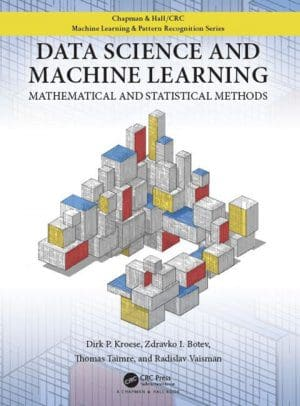# Data Science and Machine Learning: The Free eBook

Check out the newest addition to our free eBook collection, Data Science and Machine Learning: Mathematical and Statistical Methods, and start building your statistical learning foundation today.

It's been a while since we shared a free eBook with our readers, but this week we have come across another worthy entrant in the series, and wanted to share it in time for the holiday learning season (which is most definitely a thing).

Today we share Data Science and Machine Learning: Mathematical and Statistical Methods, by D.P. Kroese, Z.I. Botev, T. Taimre & R. Vaisman. The book was published last year, and aside from being freely-available as a PDF can also be purchased in print form (and Kindle).Data Science and Machine Learning: Mathematical and Statistical Methods is a practically-oriented text, with a focus on doing data science and implementing machine learning models using Python. It does a good job of explaining relevant theory and introducing the necessary math as needed, which results in very nice pacing for a practical book.

The book's raison d'être, according to its website, is actually somewhat at odds with my take:

The purpose of this book is to provide an accessible, yet comprehensive textbook intended for students interested in gaining a better understanding of the mathematics and statistics that underpin the rich variety of ideas and machine learning algorithms in data science.

I believe this is the opposite side of the same coin: where I see this book's strength as teaching the practical and reinforcing it with the necessary theory and underlying math, the argument can clearly be made that it focuses on the theory and underlying math and reinforces this with practical implementation.

Even money, I'd say.

Regardless of the approach you endorse, the book's table of contents are as follows:

1. Importing, Summarizing, and Visualizing Data
2. Statistical Learning
3. Monte Carlo Methods
4. Unsupervised Learning
5. Regression
6. Regularization and Kernel Methods
7. Classification
8. Decision Trees and Ensemble Methods
9. Deep Learning

Lots of relevant topics are covered here, and in logical succession. I particularly like the transition from Monte Carlo methods to unsupervised learning, and how that happens prior to the introduction of supervised concepts. Classification, though likely more useful in the long run (at least seemingly so at present) seemed far less impactful than did clustering when I first encountered machine learning, and so in my view its introduction prior may prove equally captivating for other new learners.

To ensure the book is self-contained for even the newest of data science and machine learning students, the book includes the adequate and useful appendices of:

1. Linear Algebra and Functional Analysis
2. Multivariate Differentiation and Optimization
3. Probability and Statistics
4. Python Primer

You won't become a complete data science expert by reading this book, but that's not its goal. By working through Data Science and Machine Learning: Mathematical and Statistical Methods you will get a solid foundation in the basics of the field, upon which more cutting edge methods and algorithms can be added.

One of my favorite machine learning books that I used as my first foray into learning the subject matter was Data Mining: Practical Machine Learning Tools and Techniques, also known as the Weka book. I really liked as a newcomer how it mixed practical and theoretical, introducing and explaining the math as needed in order to learn the practical implementation being presented at the time. I find that this book is reminiscent of that format, with the advantage of using Python instead of the Weka toolkit which, at least today, is a much more relevant implementation pathway.

I recommend this book to anyone learning the basics of data science and machine learning, and looking to do so in the presentation format described.

Related:

By subscribing you accept KDnuggets Privacy Policy

By subscribing you accept KDnuggets Privacy Policy

By subscribing you accept KDnuggets Privacy Policy